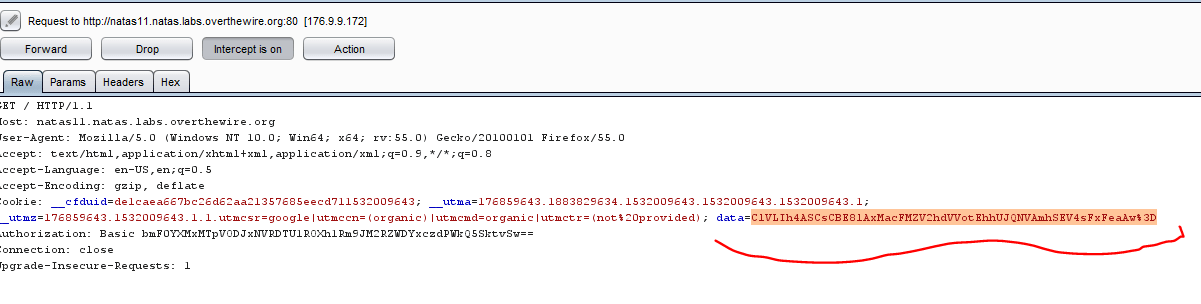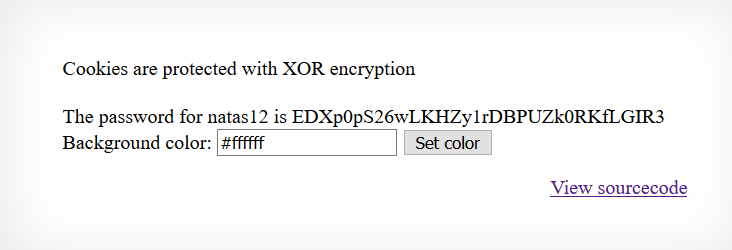LEVEL 11-12 is quite hard. It is about encryption, encoding, cookie and php code.

### Let’s dive in it:

Login with the password we found in the last article, You will see the screen below.The hint points us to the cookie, So I checked cookie using Burpsuite and there is this string I found in data variable.Let’s view the source and see what’s happening behind the scenes:#### Explanation of the code above:

In the above snippet screenshot look at the function xor_encrypt(\$in). This function is taking an input variable of a string, \$key variable is the key for XOR that we need to find. \$text is the string, the function is taking as input.

KEY xor TEXT = CIPHERTEXT
but since in this case we do not have the key but we know the text and ciphertext, we can obtain the key from them both:
CIPHERTEXT xor TEXT =KEY

Now we need to find the text which \$in variable contains. So if you look back in the code on the top there is a line \$defaultdata which contains array string. Now look at the end line \$data variable which takes the input as loadData(\$defaultdata).  The loadData is a function itself. Let’s check what loadData function is doing: it base64 decode the data, then xor_encrypt it and then json_decode it. So data is going in that form.

So we have to create a php function that takes the values in the reverse order this way and outputs the key. I have executed the code below in the online php compiler.
The code takes the actual data cookie and see the \$key value here which takes the json_encode array of the above defaultdatavariable. When we xor these two.

CODE:

``````<?php

function xor_encrypt(\$in) {
\$text = \$in;
\$outText = '';

// Iterate through each character
echo strlen(\$text)." ";
for(\$i=0;\$i<strlen(\$text);\$i++) {
\$outText .= \$text[\$i] ^ \$key[\$i % strlen(\$key)];
}

return \$outText;
}

?>  ``````In the above output 41 is the number of times it got in loop and qw8J is the XOR key which we needed. So now in the \$key value we will enter the key and \$c variable will contain the array value but will be different, the showpassword will contain yes. The xor_encrypt takes the json_encoded \$c value and then base64_encode it. Finally what we will have is the actual cookie that we need.The output here ran 42 times and the string is the cookie data that we need to submit to the website now. Once we submit it using burp suite.We now obtained the required cookie for the next level.We will continue the next level in the next post.This site uses Akismet to reduce spam. Learn how your comment data is processed.